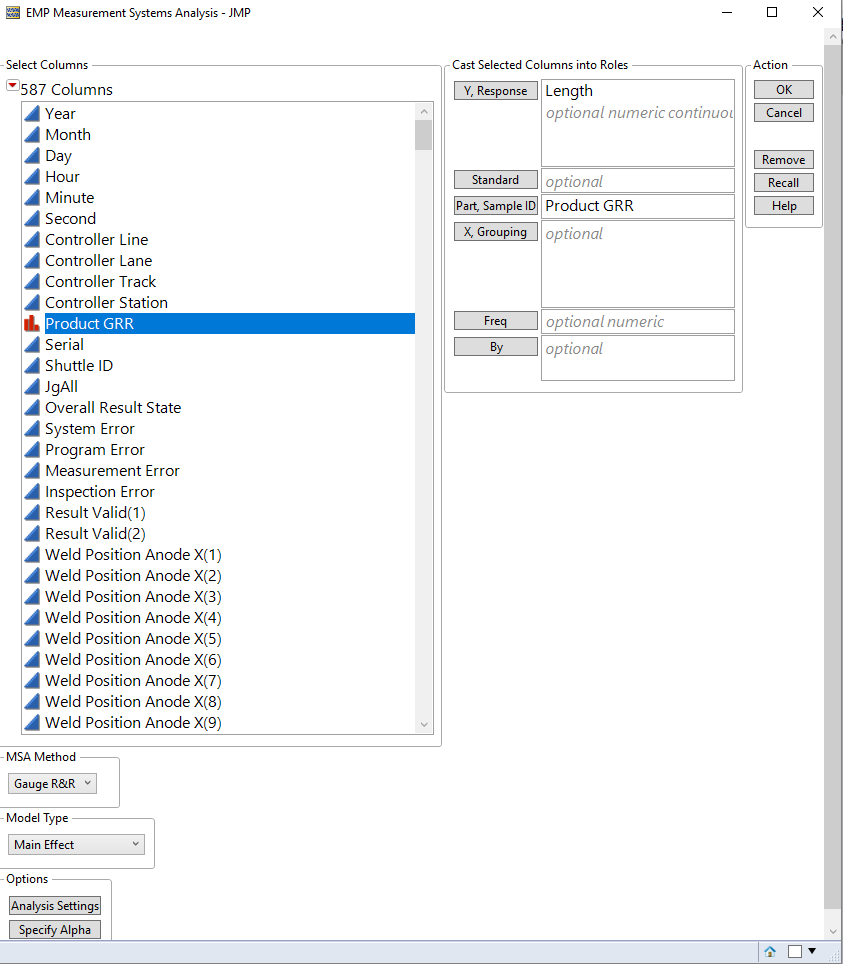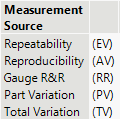Choose Language Hide Translation Bar
Highlighted

## Gage R&R Formula

Hello,

I'm looking for the detailed formula of the Gage R&R study which isn't described in the document "JMP Quality & Process Methods".

Someone knows on which basis this analysis is defined, and which formula this is?

Example: I have an optical high resolution measurement system, measuring 5 different products each 10 times in an automated cycle repetition (moving the axis forward and backwards stopping the axis in front of the measurement system, measuring, than again moving backwards, forwards, stopping in front of the measurement system, 10 times, than next product ingressed).

Using the measurement system analysis "Gauge R&R" in JMP with 6sigma as setting and a specific tolerance field USL - LSL:Thanks,

Michel

7 REPLIES 7
Highlighted

## Re: Gage R&R Formula

Michel,

Here is a link to the discussion of measurement systems analysis:

1. Your study is not exactly how a measurement study should be completed.  I may not understand your particular situation, but you should completely remove the product and re-ingress (your words) from the equipment before taking a 2nd )or 3rd, etc.) measurement.  Set-up of the product into the equipment is part of the measurement process.

2. It is extremely important that you understand how the 5 samples of product were acquired.  To determine the appropriate acquisition strategy, you need to understand what does the measurement system need to be able to discriminate.

Highlighted

## Re: Gage R&R Formula

Hello Statman,

in the link you posted, I cannot find the exact formula for the calculation of the different factors, like shown below:1. The Re-Ingress mode is correct like it is written in the description.

2. That's clear for me.

Just understanding what formula is behind the Gage R&R study.

Thanks,

Michel

Highlighted

## Re: Gage R&R Formula

There are many ways to estimate variance components.  EMP method based on Wheeler & Lyday or something more traditional.  Which method do you want to use?  For Gage R&R, see:

Highlighted

## Re: Gage R&R Formula

Sorry for that, but I cannot find the mathematical formula of the Gage R&R method used in JMP in this link.

What are the mathematical formulas behind the following analysis types:

• Gauge R&R EMS
• Gauge R&R REML
• Gauge R&R Bayesian
• Gauge R&R Anova
Highlighted

## Re: Gage R&R Formula

The formulas are given in the output itself.  See the last column of the output for the formulas.

The formula for EV is 6*sqrt(V(Within)).

You can find the details for the variance components in the documentation.

https://www.jmp.com/support/help/en/16.0/#page/jmp/statistical-details-for-variance-components.shtml...

Further information about variance components can be found in their respective chapters depending on the type of analysis you choose (EMS, REML, Bayesian, etc.)

Highlighted

## Re: Gage R&R Formula

Hello Tonya,

thanks for the reply. What does "Within" stays for in the formula of the variance of "Within" V(Within)?

Thanks,

Michel

Highlighted

## Re: Gage R&R Formula

This is the within group variation.  Here is a web page that discusses this topic.

https://www.statisticshowto.com/within-group-variation/#:~:text=Within%2Dgroup%20variation%20(someti...

Article Labels